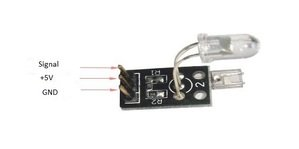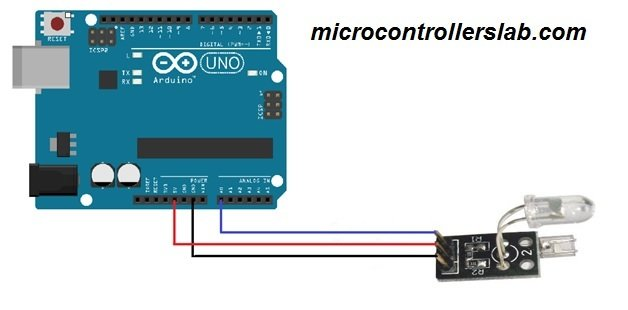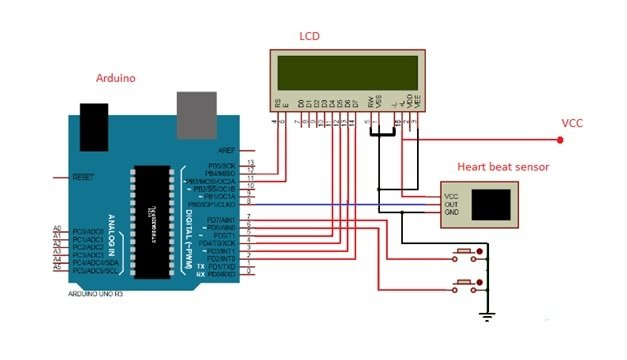# heart beat sensor with Arduino|heart pulse measurement

Heart Beat Sensor is used blood pressure and body temperature are very important parameters to known for human body. We go to doctors that use different kinds of apparatuses to know the heart beat of a human. In this tutorial, we are going to make our own heart beat sensor that will tell us the heart rate. We will make an Arduino based heart beat sensor that will tell us the number of pulses in a minute when we will place a finger on it.

### Working of Heart beat sensor

The module uses an infrared led (IR) and a photo transistor to detect the pulse of the finger and whenever a pulse is detected, red led flashes. There will be led on the light side of the finger and a photo transistor on the other side of the finger. Photo transistor is used to obtain the flux emitted. The resistance of the photo resistor will change when the pulses will change.

### Heat pulse sensor Pin Out

The heart beat sensor module has three pinsSignal: This will be connected to the analog pin of the Arduino

5V: This will be connected to the 5V pin of the Arduino

GND: This will be connected to the ground of the Arduino

### Heat beat sensor interfacing with arduinoThe connections are very easier. First connect the 5v and gnd pin of the module with the 5v and the gnd of the Arduino and then connect the sensor pin to the A0 of the Arduino.

### heart beat sensor with arduino code

This code is for the testing of heart pulse measurement.

```int led_Pin = 13;                                      // initializing the led pin

int output_Pin = A0;                                // initializing the sensor output pin

//initializng other variables

double alpha = 0.75;

int period = 200;

double change = 0.0;

void setup ( )                           // Code written in it will only run once.

{

pinMode (led_Pin, OUTPUT);                   // declaring led pin as output

Serial.begin (115200);                                   // setting baud rate at 115200

}

void loop ()

{

// initializing other variables

static double oldValue = 0;

static double oldChange = 0;

double value = alpha * oldValue + (1 - alpha) * rawValue;         // calculating values using the formula

Serial.print (rawValue);              // printing the sensor output value on the screen

Serial.print (",");

Serial.println (value);             // printing the heart beat value on the screen

oldValue = value;

delay (period);

} ```

### Heart Beat sensor with LCD attached

In this example we will attached the heart pulse sensor with Arduino and a lcd. Arduino will control the whole system. Arduino will read the pulses from the heart beat sensor module and will calculate the heart rate and will show it to the LCD.The output pin of the heart beat sensor is connected to the pin 8 of Arduino. LCD is connected to Arduino in the 4-bit mode. VCC and gnd of sensor are connected to the vcc and gnd of the Arduino. When we will press the push button the system will start to count the pulses.There are many methods for calculating the heart beat but here we will take only five pulses and will calculate the total heart rate in minute by using this formula.

Five pulse time = time2 – time1

Single pulse time = Five pulse time /5

Rate = 60000/ Single pulse time;

where time1 is first pulse counter value

time2 is list pulse counter value

rate is final heart rate

Components

The components used for making this project are as follows.

• Arduino (we have used Arduino uno)
• 16 X 2 LCD
• Heart beat sensor
• Push button

### Code of heart beat measurement

This code is for displaying the output of heart pulse sensor on the lcd. install the library of the lcd before uploading the code.

```#include<LiquidCrystal.h>                                            // including the lcd library

LiquidCrystal lcd(12, 11, 5, 4, 3, 2);                             // declaring pins for lcd

int in = 8;                                                      // declaring pin 8 for sensor output
int Reset=6;                                                   // declaring pin 6 for push button
int start=7;                                                    // declaring pin 7 for other push button
int count=0,i=0,k=0,rate=0;                                         // initializing other variables

unsigned long time2,time1;
unsigned long time;

byte heart =
{
0b00000,
0b01010,
0b11111,
0b11111,
0b11111,
0b01110,
0b00100,
0b00000
};

void setup ( )
{
lcd.createChar(1, heart);
lcd.begin(16,2);                                               // starting the lcd

lcd.print("Heart Beat ");                              // printing heart beat on the display
lcd.write(1);
lcd.setCursor(0,1);                                         // setting the cursor from start
lcd.print("Monitering");                              // printing monitoring on the display
// initializing the pins as input pins

pinMode(in, INPUT);
pinMode(Reset, INPUT);
pinMode(start, INPUT);

// setting the push buttons state as high
digitalWrite(Reset, HIGH);
digitalWrite(start, HIGH);
delay(1000);                                     // giving a delay
}

void loop()
{
if(!(digitalRead(start)))                       // checking if the start button is pressed or not
{
k=0;
lcd.clear();
while(k<5)                                     // applying another condition
{
{
if(k==0)
time1=millis();
k++;
}
}
// applying the formula for calculating the heart beat

time2=millis();
rate=time2-time1;
rate=rate/5;
rate=60000/rate;
lcd.clear();                                       // clearing the lcd
lcd.print("Heart Beat Rate:");                    // printing heart beat rate on display
lcd.setCursor(0,1);                            // setting the cursor at start
lcd.print(rate);                            // printing the heart beat on the display
lcd.print(" ");
lcd.write(1);
k=0;
rate=0;
}
if(!digitalRead(Reset))                         // checking if reset button is pressed or not
{
rate=0;
lcd.clear();                              // clearing the lcd
lcd.print("Heart Beat Rate:");          // printing heart beat rate on display
lcd.setCursor(0,1);                    // setting the cursor at start
lcd.write(1);
lcd.print(rate);                       // printing the heart beat on the display
k=0;
}
}```

### 16 thoughts on “heart beat sensor with Arduino|heart pulse measurement”

1. Hi sir i am astudent of omputer engineering .i have proposed to submit the same projec t as my senior project. but i want the system to be “an ecg based heart rate monitoring system using arduino microcontroller with the ecg graph on an android app with bluetooth technology”.So can you help me what components i need clearly.

2. sir this code is not working properly if we press pushbutton to monitor heart rate it stays at “please wait” ?????

• Hey,we have same problem of lcd showing please wait…..
What you did then.did your project run?if you got diffrent code then please send me.

3. hello sir
i use Pulse Rate Sensor Heartbeat Heart Rate
my LCD is show random number means heartbeat show wrong
i want to ask this programming is right for Pulse Rate Sensor Heartbeat Heart Rate this sense
if any prob in my project
could you tell me
i will be thank full to you

• hello sir
i use Pulse Rate Sensor Heartbeat Heart Rate
my LCD is show random number means heartbeat show wrong
i want to ask this programming is right for Pulse Rate Sensor Heartbeat Heart Rate this sense
if any prob in my project
could you tell me
i will be thank full to you

4. Buenos dias disculpe para utilizar el simulador proteus como sale el sensor

5. please give me the program for electronic stethoscope.

6. Sir we doing project on werable health monitoring for that we need code for heart beat sensor using arduino nano r3

7. why should we intialize alpha value as 0.75?

8. Can I get the Pulse displacement amplitude using this sensor?
If yes, then how ?

9. Can u plz send me the code.

• int led_Pin = 13; // initializing the led pin

int output_Pin = A0; // initializing the sensor output pin

//initializng other variables

double alpha = 0.75;

int period = 200;

double change = 0.0;

void setup ( ) // Code written in it will only run once.

{

pinMode (led_Pin, OUTPUT); // declaring led pin as output

Serial.begin (115200); // setting baud rate at 115200

}

void loop ()

{

// initializing other variables

static double oldValue = 0;

static double oldChange = 0;

double value = alpha * oldValue + (1 – alpha) * rawValue; // calculating values using the formula

Serial.print (rawValue); // printing the sensor output value on the screen

Serial.print (“,”);

Serial.println (value); // printing the heart beat value on the screen

oldValue = value;

delay (period);

}